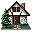Home Existing users log in here or new users sign up here. Kinematics Puzzles Mechanics Puzzles Electric Circuits Puzzles Magnetism Puzzles Kinematics Surprising Data Mechanics Surprising Data Electrostatics Surprising Data Fluids Surprising Data Optics Surprising Data

### How far was the ball launched? Challenging version: Application Experiment

#### Aim

Measure how far the ball travelled for two cases (30 degrees and 45 degrees) using two independent methods

#### Prior Knowledge

Projectile motion

#### Description of the Experiment

A ball is launched at two angles, first at 30 degrees then at 45 degrees. In each case, two trials are conducted to reduce experimental uncertainty. Use the videos to make appropriate measurements to estimate how far the ball flew. Make the estimates relative to the sill of the blackboard. You need to come up with two independent methods to measure the distance and compare your answers at the end.

For each method make explicit the following:

• The model you are going to use to estimate the distance,
• the assumptions you are making,
• how these assumptions might affect your estimates.
You should also make estimates of the uncertainties in each estimate and, at the end, compare your answers and try to explain any discrepancies you might find.